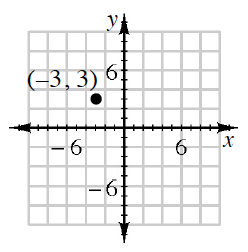### Home > INT1 > Chapter 6 > Lesson 6.2.2 > Problem6-62

6-62.

Yesterday Mica was given some information and was asked to write a linear equation. But last night her cat destroyed most of the information! At right is all she has left:

1. Complete the table and graph the line that represents Mica’s rule.

Because the equation is linear, the growth must be constant.

Use the points below to graph the line represented by Mica's chart.

2. Mica thinks the equation for this graph could be $2x + y = −3$. Is she correct? Explain why or why not. If not, write your own equation to match the graph and $x → y$ table.

Put the equation into $y = mx + b$ form and see if the growth rate and $y$-intercept match the line you graphed in part (a).$x$

$y$

$-3$

$-2$

$1$

$-1$

$0$

$1$

$2$

$3$

Use the eTool below to help solve the problem.
Click the link at right for the full version of the eTool: 6-62 HW eTool